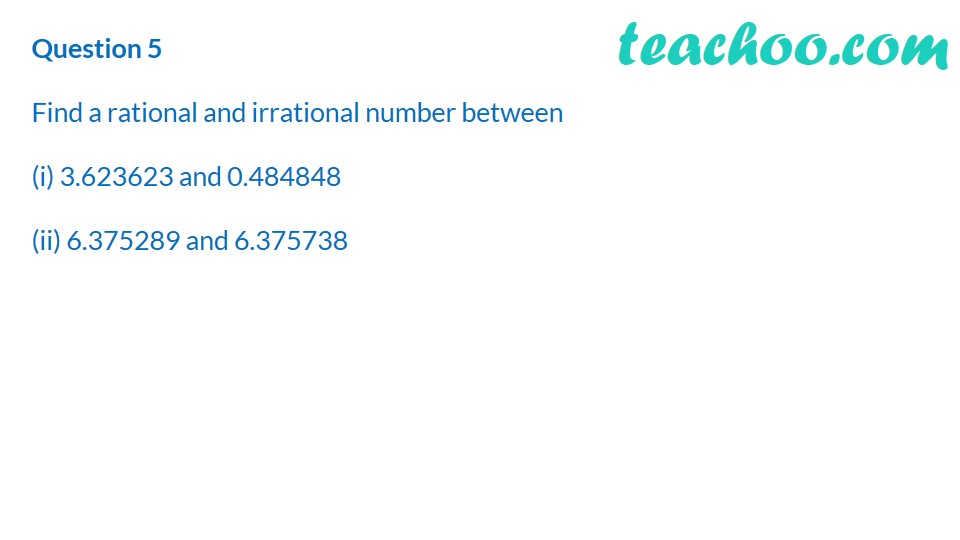Learn All Concepts of Chapter 1 Class 9 - FREE. Check - Chapter 1 Class 9 Maths1. Chapter 1 Class 9 Number Systems
2. Concept wise
3. Finding irrational numbers b/w two numbers

Transcript

Question 5 Find a rational and irrational number between (i) ﻿3.623623 and 0.484848 (ii) ﻿6.375289 and 6.375738

Finding irrational numbers b/w two numbers